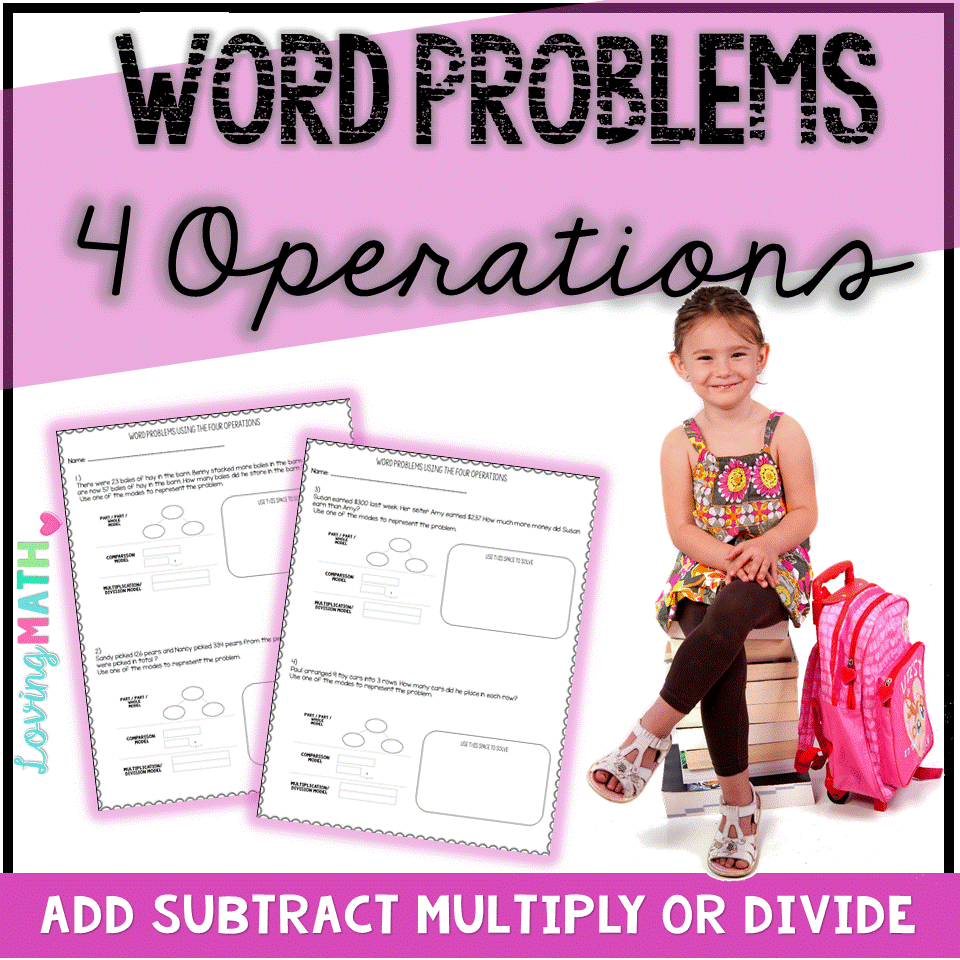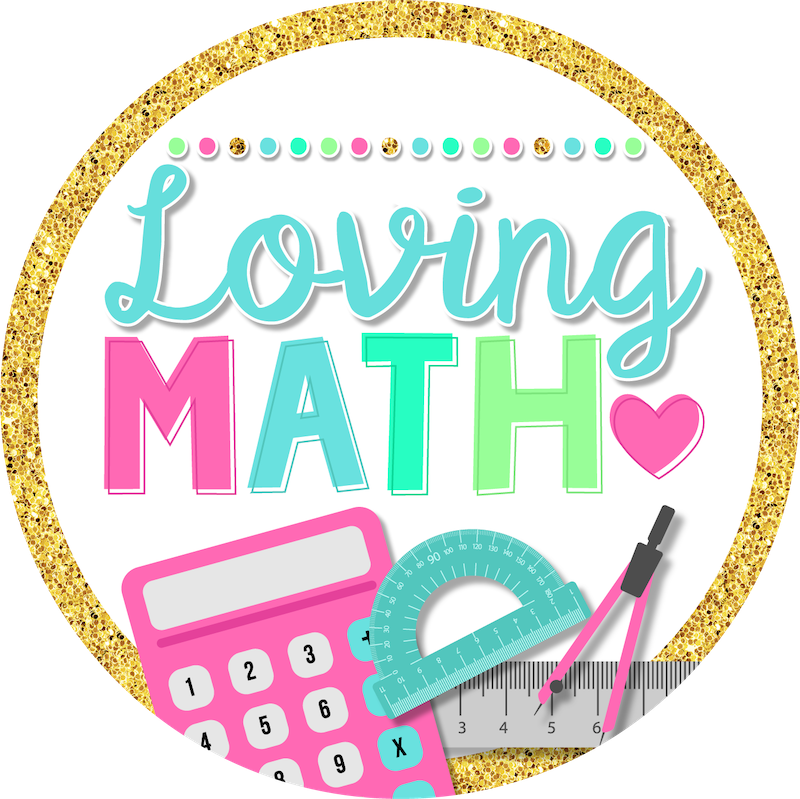# WORD PROBLEM STRATEGIES FOR DECIDING WHICH OPERATION TO PERFORM

In my years teaching students and providing support to other teachers, one of the biggest challenges I’ve encountered has been solving word problems. Most students are able to compute but are often puzzled by which operation to perform. In fact, that is usually the first question they ask after they read the word problem.

“Is this subtraction?”

“Is this multiplication?”

“Is this division”?

To be honest, the way we teach math is mostly to blame. Think about most math textbooks. There is often a unit on addition (or subtraction, or multiplication, or division), followed by a problem solving lesson at the very end. Kids know they are learning that skill so they don’t have to think about the operation needed. Tests are designed the same way. A chapter test on Addition. Well, no wonder our kids don’t have the strategies to decide which operation is needed when they are presented with all these word problems at once.

Other teachers rely on clue words. In my humble opinion, this is not enough and is often misleading.

For example:

the word total can be any operation

the word each could be multiplication or division

the word left could be subtraction, or addition

Sometimes no clue words are mentioned at all. What are kids to do?

I have created some lessons to help kids decide which operation is needed when solving one step problems. As a math coach,  have tried these lessons with our third grade students and have seen considerable increase in understanding.

For addition / subtraction problems, we teach them various scenarios that call for addition and subtraction. We then, break up those problems into two categories:

• PART PART WHOLE problems
• COMPARISON problems

Students then, are presented with models for each type of problem and graphic organizers to help them organize their thinking.

A variety of practice problems are shared with students, where they used the graphic organizers given to decide which operation is needed to solve.

Multiplication and Division word problems are taught the same way:
Strategies are shared on how to decide when a problem calls for multiplication or division.
Do you want to know what the third grade students shared with me when I asked them how do they decide if they need to multiply or divide?  These are some of the answers I received:
• “I know I need to multiply when the numbers are small like 3 and 6.”
• ” I divide when they give me a big number and a small number, like 64 and 8.”
• “I multiply when it says each.”
• “I divide when it says share equally.”
Yup, my mouth dropped. But when I though about it, I understood why they though like this. This is how most textbooks present these word problems in third grade. No wonder, when they numbers get larger they get really confused.
This is how we solved that problem:
And they were presented with multiple opportunities to practice using this model.
If you would like to try these lessons with your students, they are listed in my TpT store.
Also, here is a FREEBIE just for you! It includes mixed word problems. All four operations are included. Hope you find it helpful.Happy Teaching!